Download the eSaral app and start learning from Kota's top IITians and doctors.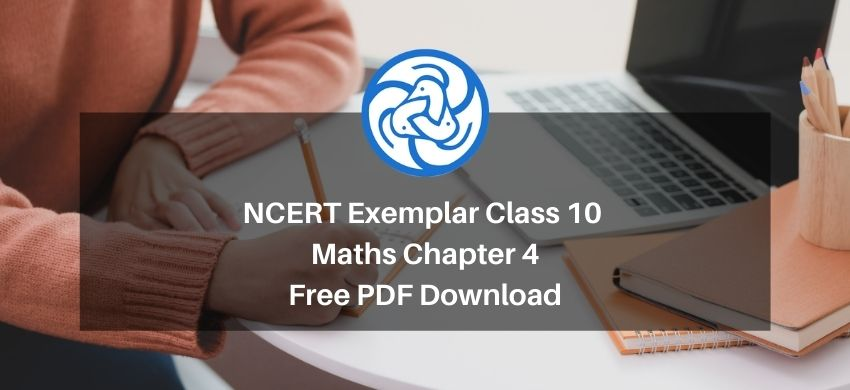Hey, are you a class 10 student? and looking for ways to download NCERT Exemplar Class 10 Maths Chapter 4 "Quadratic equations"? If yes. Then read this post till the end.

If you want to score high in your Class 10 Maths exam then it is very important for you to understand and learn the topics of Class 10 Maths thoroughly.

So, without wasting more time let’s start.

### Download The PDF of NCERT Exemplars Class 10 Maths Chapter 4 "Quadratic equations"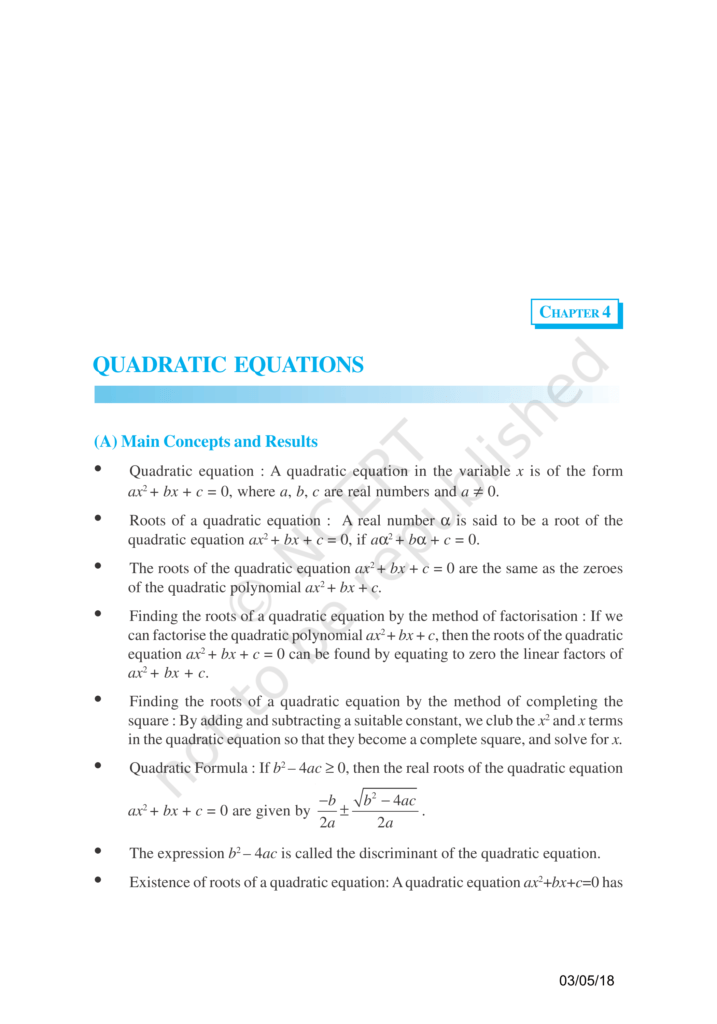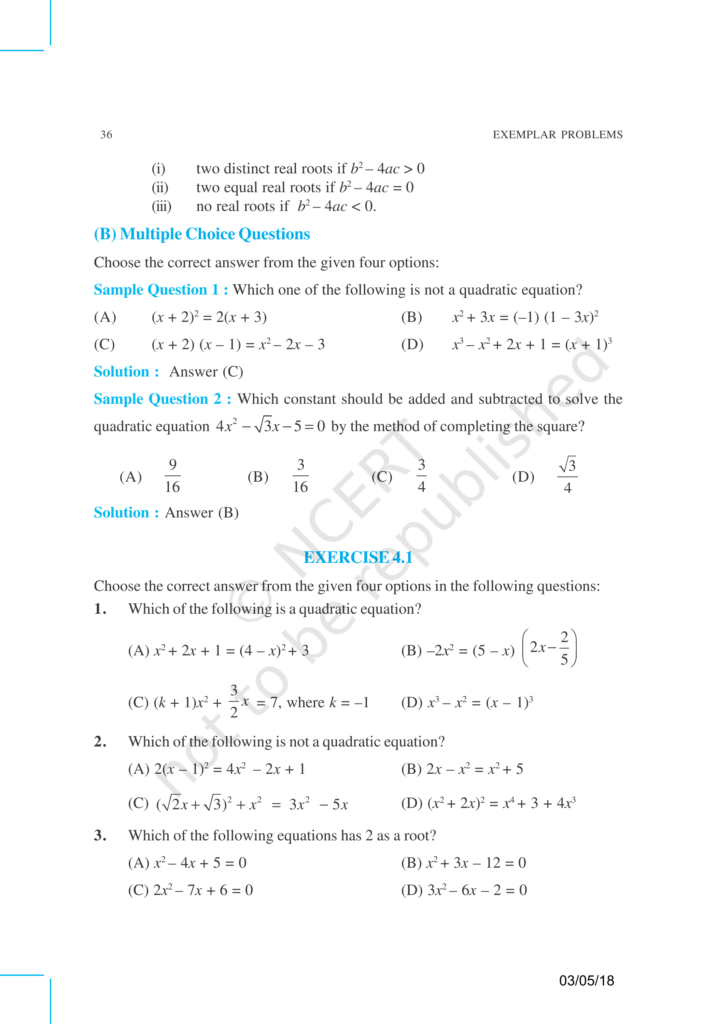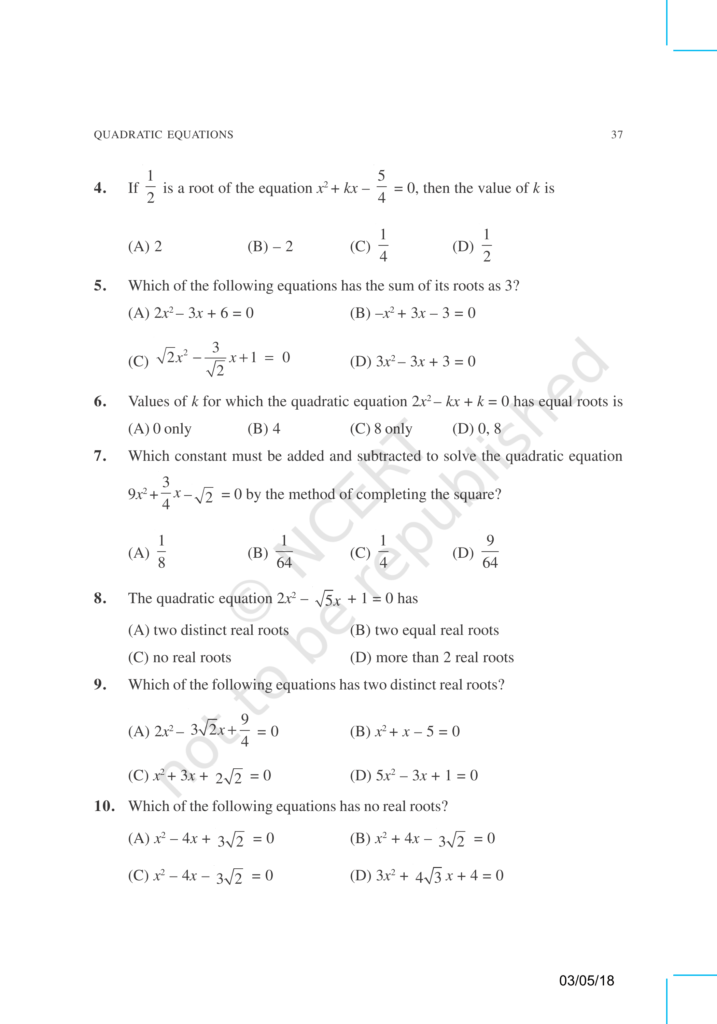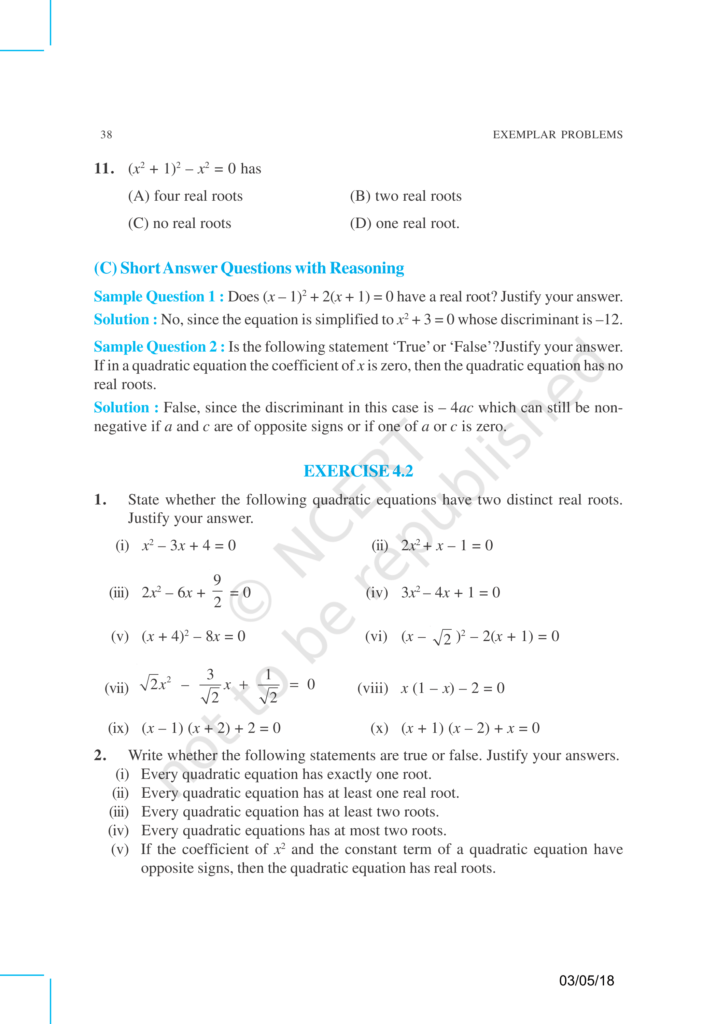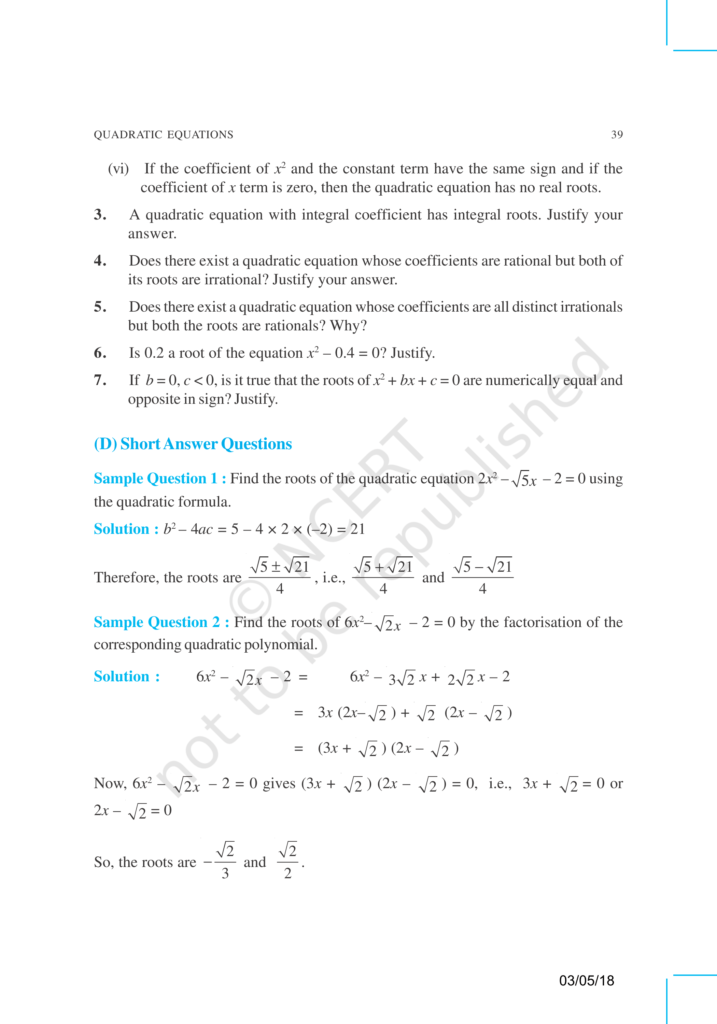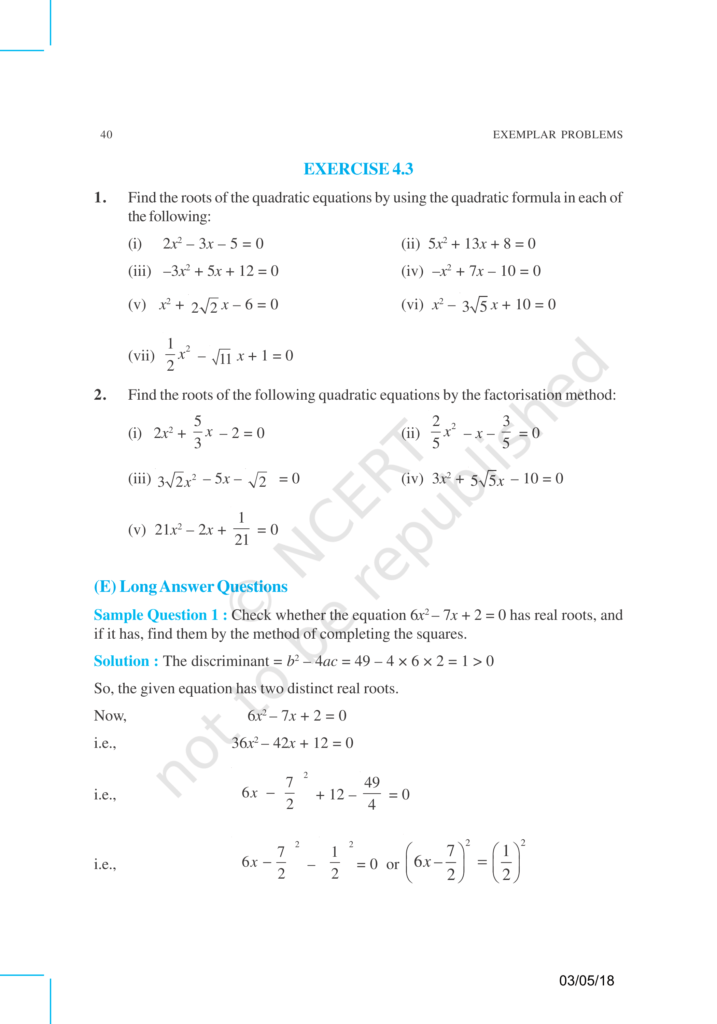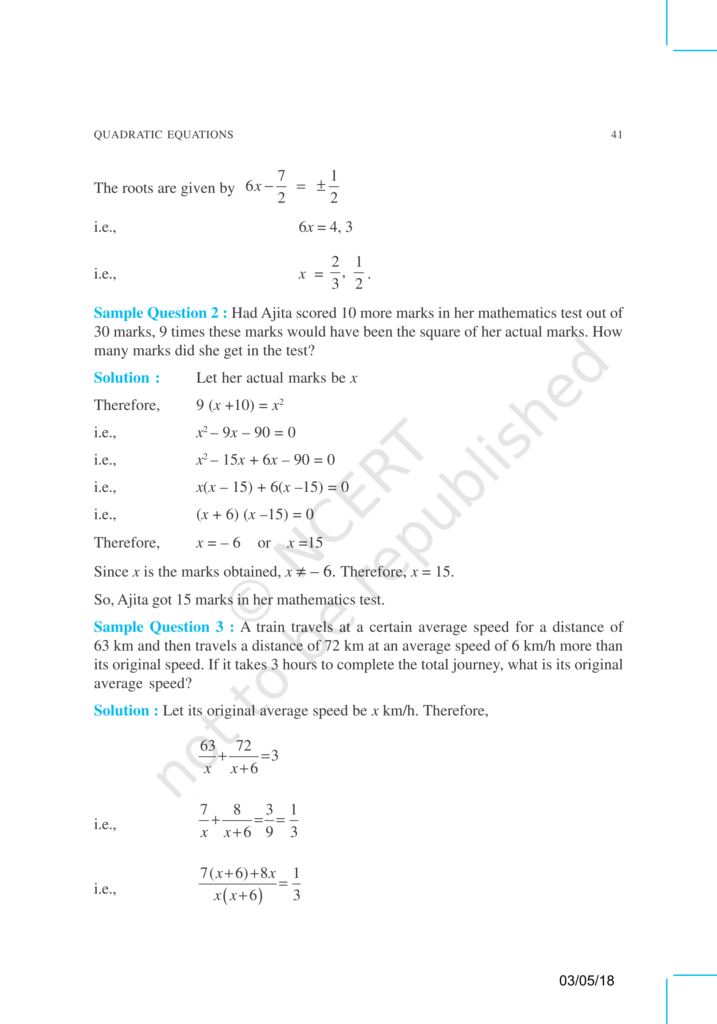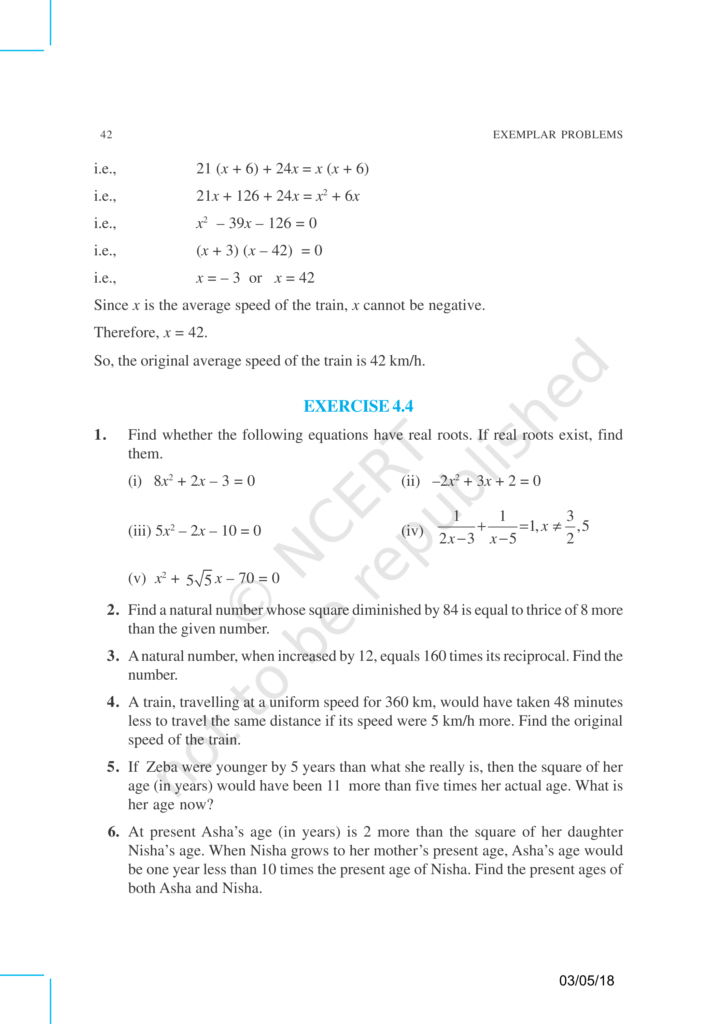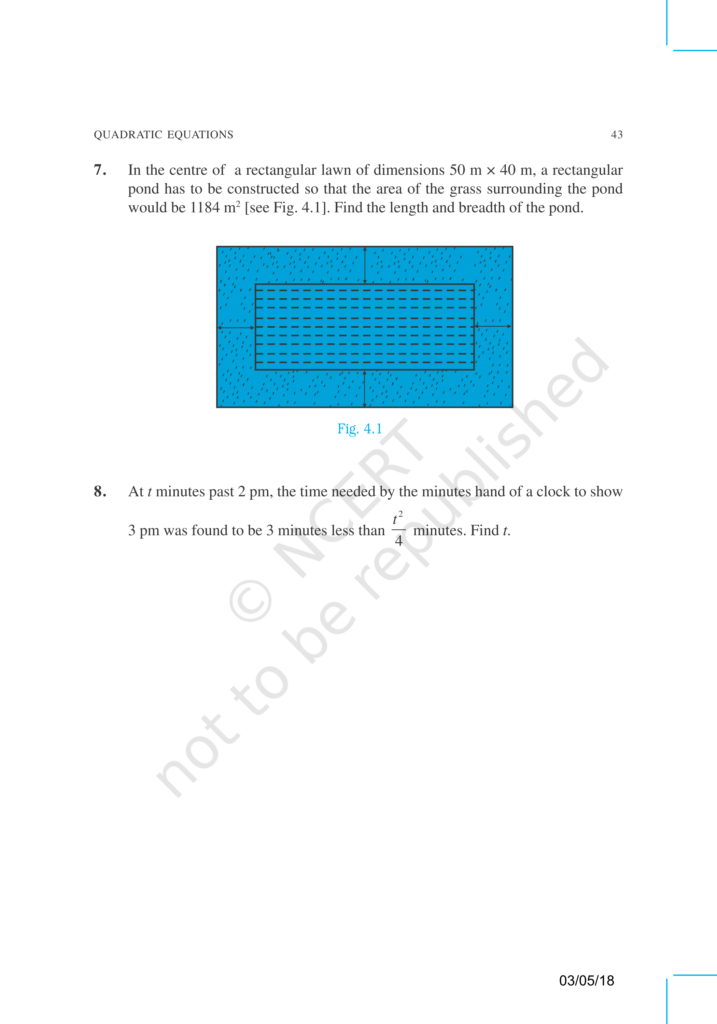Class 10 Maths Revision Notes

NCERT Class 10 Maths Book

CBSE Class 10 Maths Syllabus

NCERT Exemplar Class 10 Science all chapters

NCERT Exemplar Class 10 Maths all chapter

NCERT Solutions for Class 10 Science

NCERT Solutions for Class 10 Maths

If you have any Confusion related to NCERT Exemplar Class 10 Maths Chapter 4 then feel free to ask in the comments section down below.

To watch Free Learning Videos on Class 10 Maths by Kota’s top Faculties Install the eSaral App